# Finding the Slope of a LineHey, guys! Welcome to this video on finding the slope!

So, I’m assuming that you already know how to find the slope of a line when given the equation of a line.

We know that the standard form for the equation of a line is $$y=mx + b$$, where $$m$$ is our slope and $$b$$ is our $$y$$-intercept.

We also know that slope is rise over run, or $$y$$ over $$x$$.

$$\text{slope}=$$ $$\frac{\text{rise}}{\text{run}}$$ $$=$$ $$\frac{y}{x}$$

But now, how do we find the slope of a line when only given two points on a graph?

Let’s take a look. So, we have our graph.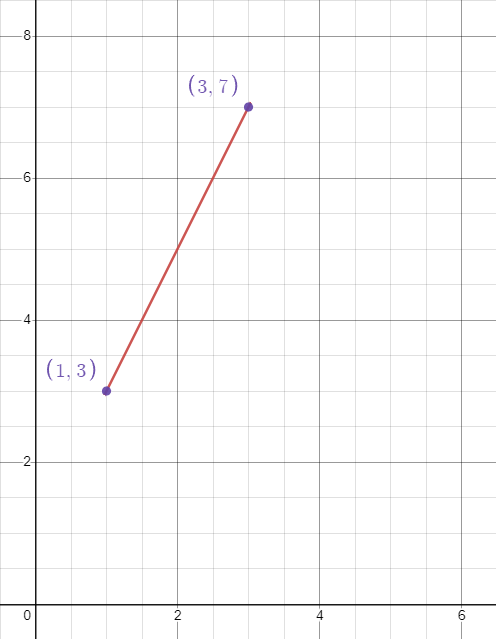So, we have two points on our line $$(1,3)$$ and $$(3,7)$$, but how do we find the slope of the line?

Well, we can do this by dividing the difference of the $$y$$-coordinates of the two points you’ve been given by the difference of the $$x$$-coordinates from the same set of points.

Let me just write out, mathematically, everything that I just said.

So, we find the slope by dividing by the difference between our $$y$$-coordinates. That can be written like this: $$\frac{y_{2}-y_{1}}{x_{2}-x_{1}}=\text{slope}$$

Now, you just need to plug in your $$y$$-values and your $$x$$-values.

But here’s another very important thing that many students get confused about, and it’s a great question: Which set of coordinates are my $$x_{1}$$ and $$y_{1}$$, and which set of coordinates are my $$x_{2}$$ and $$y_{2}$$?

The answer is: It doesn’t matter.

However, what you can’t do is assign $$x_{1}$$ to the $$x$$-value in a set of coordinates, and $$y_{2}$$ to the corresponding $$y$$-values. For example, in our points on the graph above, I couldn’t say that the 1 is my $$x_{1}$$ and then the 3 is my $$y_{2}$$. If I make 1 $$x_{1}$$, then my corresponding $$y$$-value here has to be $$y_{1}$$. What doesn’t matter is whether or not I make this set of points my $$(x_{1},y_{1})$$, or this set of points my $$(x_{1},y_{1})$$.

So now, let’s pick whichever point we want to be our $$(x_{1},y_{1})$$ and for this video I’ll just say that $$(1,3)$$ will be our $$(x_{1},y_{1})$$, which makes $$(3,7)$$ our $$(x_{2},y_{2})$$.

Now, let’s plug in our values:

Since 7 is our $$y_{2}$$, we have $$7-y_{1}$$, and our $$y_{1}$$ is equal to 3. So, $$7-3$$. Over the difference between our $$x$$-values, $$x_{2}$$, which is 3 minus $$x_{1}$$, which is 1.

So we have $$\frac{7-3}{3-1}$$. That’s gonna give us $$\frac{4}{2}$$, which reduces to be $$\frac{2}{1}$$, which is the same thing as 2.

Just to show you guys it doesn’t matter which set of points you make your $$(x_{1},y_{1})$$, and $$(x_{2},y_{2})$$, I’ll switch them around.

So let’s make $$(1,3)$$ $$(x_{2},y_{2})$$ and $$(3,7)$$ $$(x_{1},y_{1})$$.

When we plug them in we get $$\frac{3-7}{1-3}=\frac{-4}{-2}$$. When we have a negative divided by a negative you get a positive, so this simply reduces to $$\frac{2}{1}$$, or 2.

So, we see that our two answers are the same, and that it does not matter which set of coordinates we assign to be our $$(x_{1},y_{1})$$, and $$(x_{2},y_{2})$$.

I hope this video has been helpful to you!

See you next time!

## Practice Questions

Question #1:

In the standard equation of a line, what do the variables m and b represent in $$y=mx+b$$?

m represents y-intercept and b represents the slope

m represents slope and b represents the x-intercept

m represents slope and b represents the y-intercept

m represents x-intercept and b represents the slope

The correct answer is m represents slope and b represents the y-intercept. In the equation $$y=mx+b$$, x and y represent a coordinate pair $$(x, y)$$ that is located on the line of the equation. The variable m represents the slope of the line, and b represents the y-intercept, or where the line crosses through the y-axis.

Question #2:

What is the slope of the line below?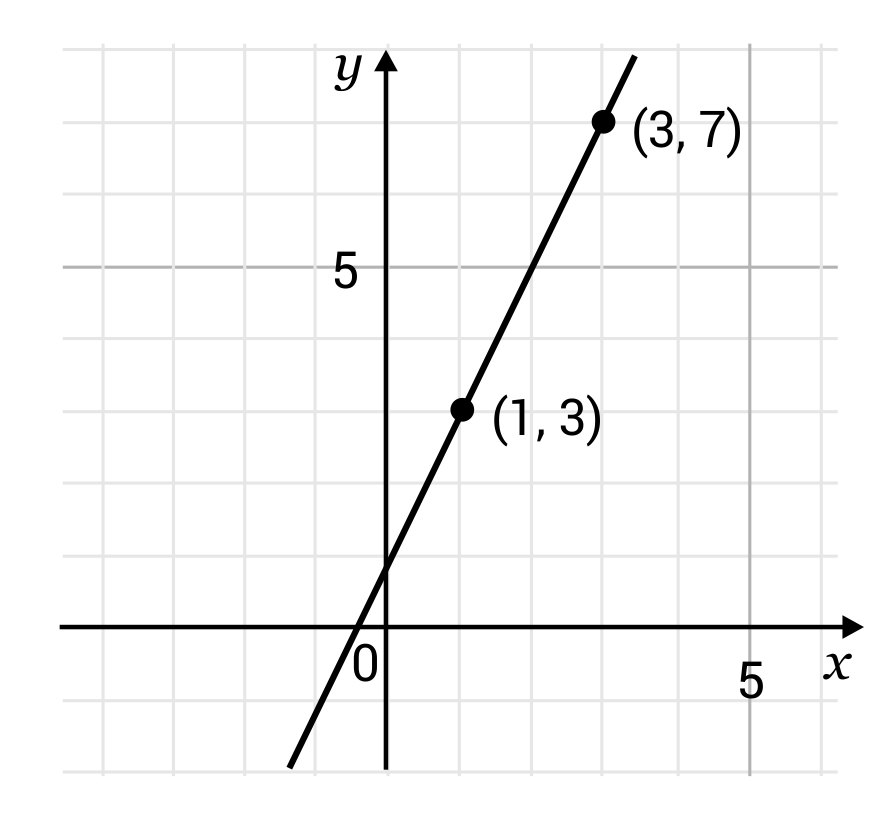$$\frac{1}{2}$$

1

2

3

The correct answer is 2. The slope of the graphed line can be found by using the two plotted points $$(1, 3)$$ and $$(3, 7)$$. The slope can be calculated by dividing the difference of the y-coordinates by the difference of the x-coordinates. Plug in the x and y coordinates:
$$y-y$$ would be $$3-7=-4$$
$$x-x$$ would be $$1-3=-2$$
$$\frac{-4}{-2}=2$$

The slope of the line, therefore, is 2.

Question #3:

What is the slope of the line below?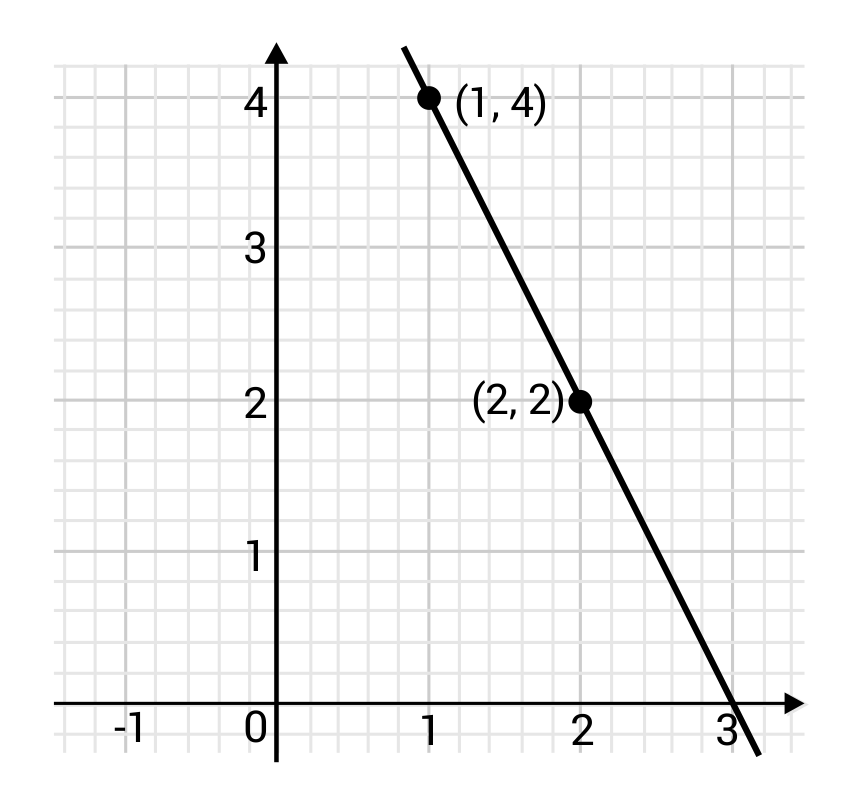-2

2

-4

4

The correct answer is -2. The slope of the graphed line can be found using the two plotted points $$(1, 4)$$ and $$(2, 2)$$. Calculate the slope by dividing the difference of the y-coordinates by the difference of the x-coordinates. Plug in the x and y coordinates:
$$y-y$$ would be $$4-2=2$$
$$x-x$$ would be $$1-2=-1$$
$$\frac{2}{-1}=-2$$

The slope of the line, therefore, is -2.

Question #4:

What is the slope of the line below?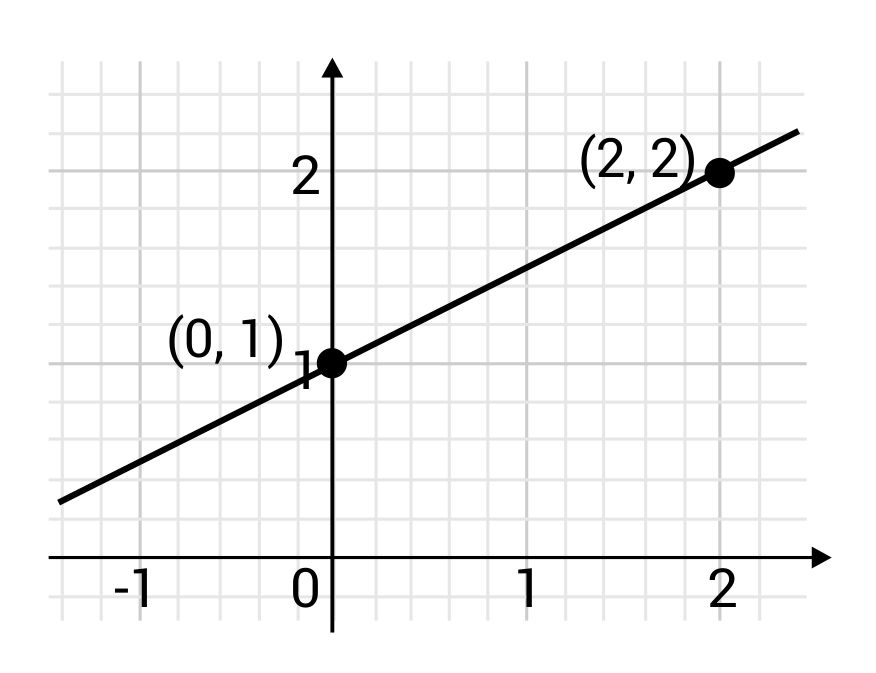$$\frac{1}{4}$$
$$\frac{1}{2}$$

2

4

The correct answer is $$\frac{1}{2}$$. The slope of the graphed line can be found using the two plotted points $$(0, 1)$$ and $$(2, 2)$$. The slope can be calculated by dividing the difference of the y-coordinates by the difference of the x-coordinates. Plug in the x and y coordinates:
$$y-y$$ would be $$1-2=-1$$
$$x-x$$ would be $$0-2=-2$$
$$\frac{-1}{-2}=\frac{1}{2}$$

The slope of the line, therefore, is $$\frac{1}{2}$$.

Question #5:

What is the slope of the line below?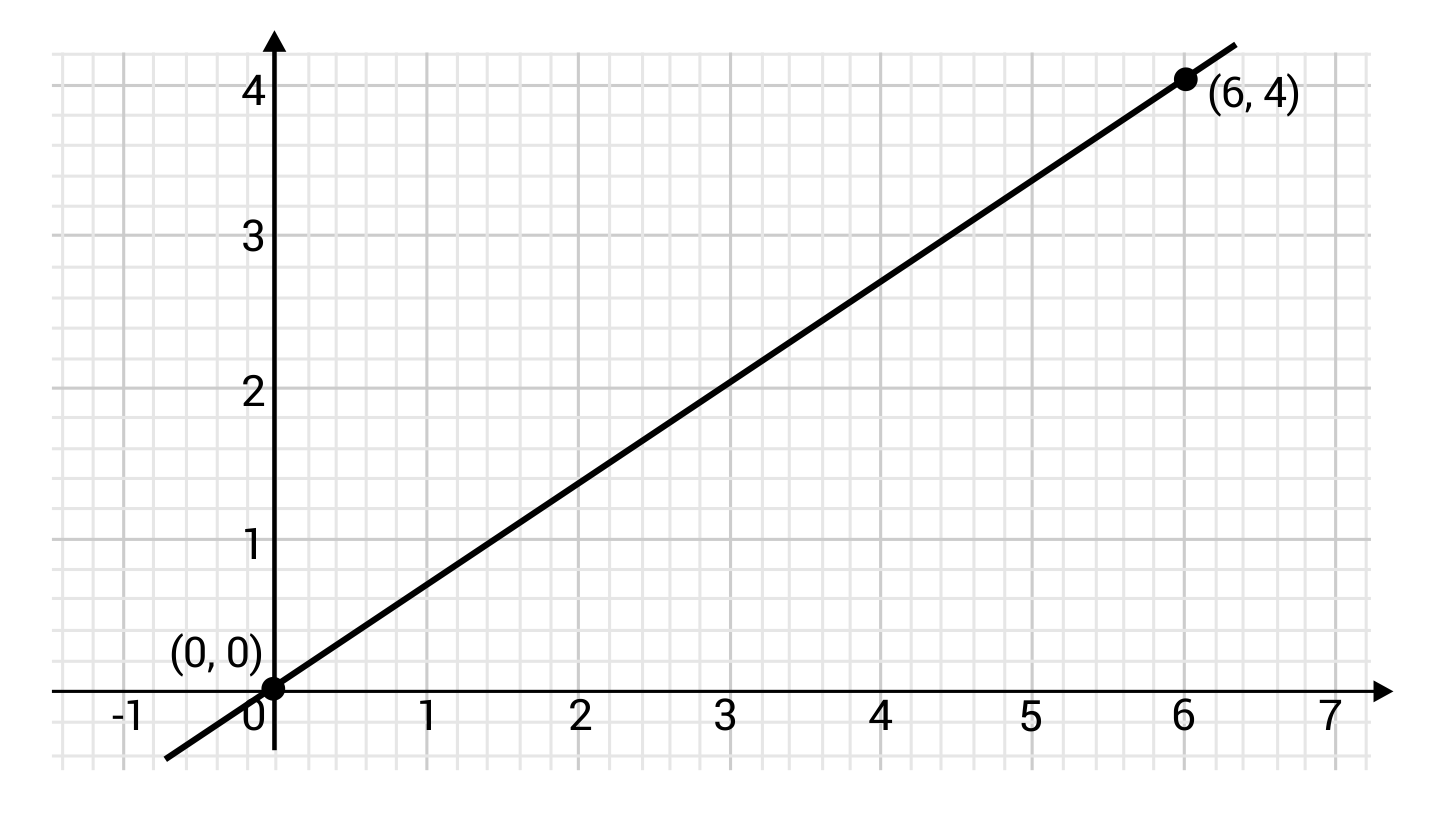2

3

$$\frac{3}{2}$$
$$\frac{2}{3}$$

The correct answer is $$\frac{2}{3}$$. The slope of the graphed line can be found using the two plotted points $$(0,0)$$ and $$(6,4)$$. The slope can be calculated by dividing the difference of the y-coordinates by the difference of the x-coordinates. Plug in the x and y coordinates:
$$y-y$$ would be $$0-4=-4$$
$$x-x$$ would be $$0-6=-6$$
$$\frac{-4}{-6}=\frac{4}{6}=\frac{2}{3}$$
The slope of the line, therefore, is $$\frac{2}{3}$$.